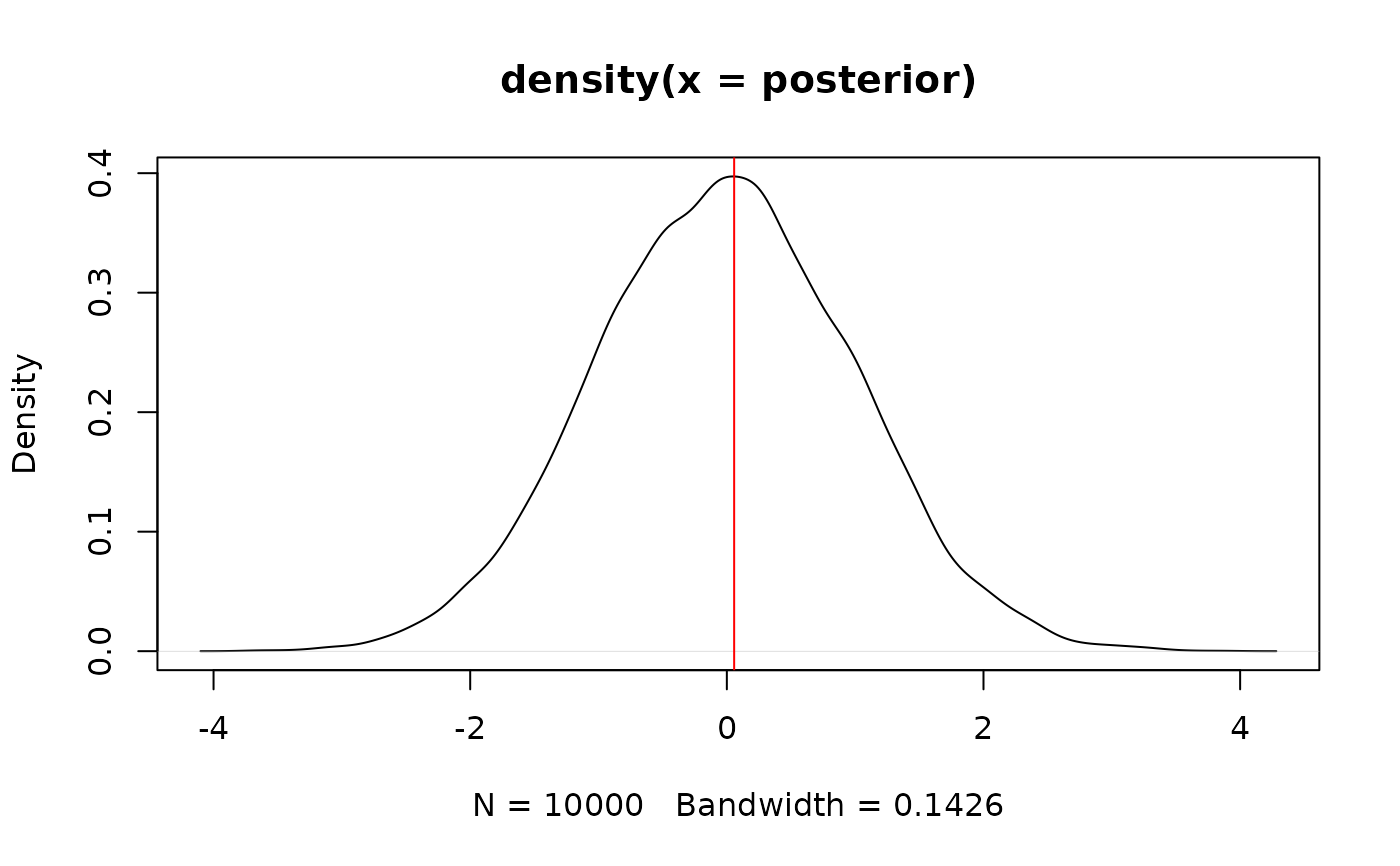Find the Highest Maximum A Posteriori probability estimate (MAP) of a posterior, i.e., the value associated with the highest probability density (the "peak" of the posterior distribution). In other words, it is an estimation of the mode for continuous parameters. Note that this function relies on estimate_density, which by default uses a different smoothing bandwidth ("SJ") compared to the legacy default implemented the base R density function ("nrd0").

map_estimate(x, precision = 2^10, method = "kernel", ...)

# S3 method for numeric
map_estimate(x, precision = 2^10, method = "kernel", ...)

# S3 method for stanreg
map_estimate(
x,
precision = 2^10,
method = "kernel",
effects = c("fixed", "random", "all"),
parameters = NULL,
...
)

# S3 method for brmsfit
map_estimate(
x,
precision = 2^10,
method = "kernel",
effects = c("fixed", "random", "all"),
component = c("conditional", "zi", "zero_inflated", "all"),
parameters = NULL,
...
)

## Arguments

x Vector representing a posterior distribution. Can also be a stanreg, brmsfit or a BayesFactor model. Number of points of density data. See the n parameter in density. Density estimation method. Can be "kernel" (default), "logspline" or "KernSmooth". Currently not used. Should results for fixed effects, random effects or both be returned? Only applies to mixed models. May be abbreviated. Regular expression pattern that describes the parameters that should be returned. Meta-parameters (like lp__ or prior_) are filtered by default, so only parameters that typically appear in the summary() are returned. Use parameters to select specific parameters for the output. Should results for all parameters, parameters for the conditional model or the zero-inflated part of the model be returned? May be abbreviated. Only applies to brms-models.

## Value

A numeric value if posterior is a vector. If posterior is a model-object, returns a data frame with following columns:

• Parameter The model parameter(s), if x is a model-object. If x is a vector, this column is missing.

• MAP_Estimate The MAP estimate for the posterior or each model parameter.

## Examples

library(bayestestR)

posterior <- rnorm(10000)
map_estimate(posterior)#> MAP = 0.02
plot(density(posterior))abline(v = map_estimate(posterior), col = "red")if (FALSE) {
library(rstanarm)
model <- rstanarm::stan_glm(mpg ~ wt + cyl, data = mtcars)
map_estimate(model)

library(brms)
model <- brms::brm(mpg ~ wt + cyl, data = mtcars)
map_estimate(model)
}